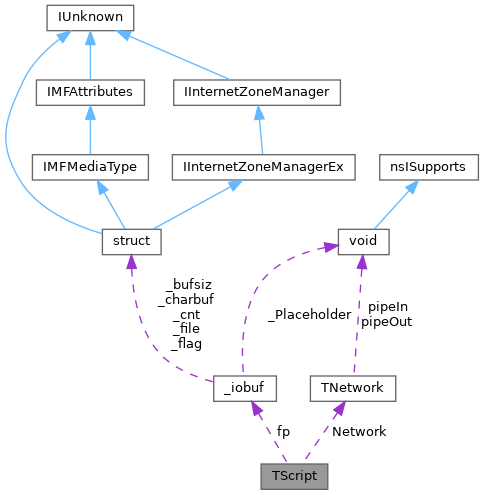ReactOS  0.4.14-dev-41-g31d7680
TScript Class Reference

#include <tscript.h>

Collaboration diagram for TScript:[legend]

Public Member Functions

TScript (TNetwork &RefNetwork)

~TScript ()

BOOL processScript (char *data)

void initScript (char *filename)

FILEfp

charscript

TNetworkNetwork

Detailed Description

Definition at line 8 of file tscript.h.

◆ TScript()

 TScript::TScript ( TNetwork & RefNetwork )
inline

Definition at line 10 of file tscript.h.

10 :Network(RefNetwork) {fp = NULL;}
TNetwork & Network
Definition: tscript.h:17
smooth NULL
Definition: ftsmooth.c:416
FILE * fp
Definition: tscript.h:15

◆ ~TScript()

 TScript::~TScript ( )
inline

Definition at line 11 of file tscript.h.

11 {}

◆ initScript()

 void TScript::initScript ( char * filename )

Definition at line 61 of file tscript.cpp.

61  {
62  if(fp) fclose(fp);
63  fp = fopen(filename, "rt");
64 }
const char * filename
Definition: ioapi.h:135
_Check_return_opt_ _CRTIMP int __cdecl fclose(_Inout_ FILE *_File)
_Check_return_ _CRTIMP FILE *__cdecl fopen(_In_z_ const char *_Filename, _In_z_ const char *_Mode)
FILE * fp
Definition: tscript.h:15

◆ processScript()

 BOOL TScript::processScript ( char * data )

Definition at line 37 of file tscript.cpp.

37  {
38 /* char* end = strchr(script,TERMINATOR);
39  if (0 == end) {
40  return true;
41  } else {
42  char* current = new char(sizeof(char)*strlen(script));
43  strncpy(current,script,(int)(end-script));
44  current[(int)(end-script)]=0;
45  char *ptr=end;
46  if (strstr(data,current) != 0) {
47  script = ++end;
48  end = strchr(script,TERMINATOR);
49  while ((ptr = strchr(ptr,SPACE_HOLDER)) != 0 && ptr < end) {
50  *ptr=' ';
51  }
52  Network.WriteString(script,(int)(end-script));
53  Network.WriteString("\r\n",2);
54  script = ++end;
55  }
56  delete current;
57  }*/
58  return TRUE;
59 }
#define TRUE
Definition: types.h:120

◆ fp

 FILE* TScript::fp
private

Definition at line 15 of file tscript.h.

Referenced by initScript(), and TScript().

◆ Network

 TNetwork& TScript::Network
private

Definition at line 17 of file tscript.h.

◆ script

 char* TScript::script
private

Definition at line 16 of file tscript.h.

The documentation for this class was generated from the following files: